# 1 Introduction

A viral quasispecies is understood as a collection of closely related viral genomes produced by viruses with low replication fidelity. RNA viruses show a high replication error rate due to a lack of proofreading mechanisms. It is estimated that for viruses with typically high replicative loads, every possible point mutation and many double mutations are generated with each viral replication cycle, and these may be present within the population at any time. Given this inherent dynamic, we may be interested in comparing the viral diversity indices between sequential samples from a single patient or between samples from groups of patients. These comparisons can provide information on the patient’s clinical progression or the appropriateness of a given treatment.

QSUtils is a package intended for use with quasispecies amplicon data obtained by NGS, but it could also be useful for analyzing 16S/18S ribosomal-based metagenomics or tumor genetic diversity by amplicons.

In this tutorial, we illustrate how the functions provided in the package can be used to simulate quasispecies data. This implies simulation of closely related genomes, (eventually with segregating subpopulations at higher genetic distances) and their abundances.

In particular, we can differentiate between acute and chronic infection profiles by the quasispecies composition. An acute infection is expected to show a prominent genome that is highly abundant, together with a set of low-abundance genomes. On the other hand, besides the implicit dynamics, a chronic infection is expected to show a number of relatively abundant genomes together with a myriad of derived genomes at low and very low abundances.

In viral terms, the fitness of a genome is a measure of its replicative performance. High-fitness haplotypes show high abundances after a transient state, during which they overcome other genomes in the quasispecies. During infection of a human host, the quasispecies typically shows variations in the fitness of each genome caused by changes in the bioenvironment. Because of this dynamic, we may observe the profile of a typical acute infection also in chronic patients, at least at the level of magnification provided by current NGS technology.

A few functions in the package were designed to simulate quasispecies composition, with the aim of studying the statistical properties of the diversity indices (Gregori et al. 2016) (Gregori et al. 2014).

# 2 Install package

BiocManager::install("QSutils")
## Warning: package(s) not installed when version(s) same as or greater than current; use
##   force = TRUE to re-install: 'QSutils'
library(QSutils)

# 3 Abundance

Two different types of information define the quasispecies composition: the genomes present and their current frequency (abundance) in the viral population. The package provides three ways to simulate abundance with various decreasing profiles.

## 3.1 Powers of a fraction

$$fn.ab$$ setting the argument $$fn$$ to $$pf$$ computes consecutive fractions, given the frequency of the most abundant haplotype, according to:

$h ~r^{(i-1)}, ~~ r<1, ~~ i=1..n$ Using $$table$$ on the $$fn.ab$$ result, we obtain the number of haplotypes for each frequency:

table(fn.ab(25,fn="pf"))
##
##     1     2     4     9    19    39    78   156   312   625  1250  2500  5000
##    12     1     1     1     1     1     1     1     1     1     1     1     1
## 10000
##     1
par(mfrow=c(1,2))
plot(fn.ab(25,fn="pf"),type="h")
plot(fn.ab(25,r=0.7,fn="pf"),type="h")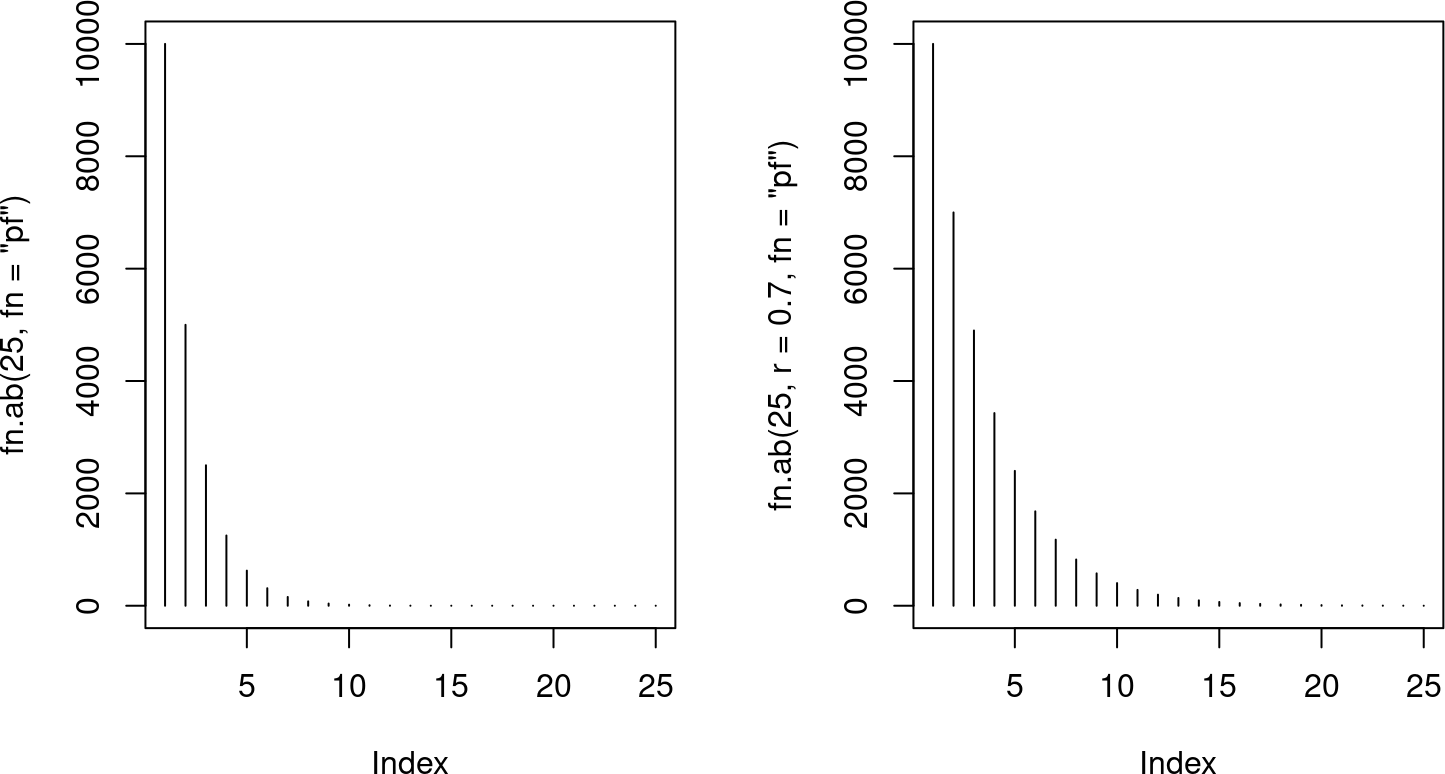Figure 1: Profile of abundances simulated with fn.ab with fn=pf

The default value for $$r$$ is 0.5. Higher $$r$$ values moderate the decrease of abundances. By default, the frequency of the most abundant haplotype, $$h$$, is 10000.

## 3.2 Power of consecutive fractions

$$fn.ab$$ with the argument $$fn="pcf"$$ computes the power of consecutive fractions, according to:

$h ~ \frac{1}{i^{r}}, ~~ r>0, ~~ i=1..n$ Default values are 0.5 for $$r$$ and 10,000 for $$h$$. The higher the $$r$$, the more pronounced the decrease in frequencies.

table(fn.ab(25,r=3,fn="pcf"))
##
##     1     2     3     4     5     7    10    13    19    29    46    80   156
##     8     3     1     1     1     1     1     1     1     1     1     1     1
##   370  1250 10000
##     1     1     1
table(fn.ab(25,r=2,fn="pcf"))
##
##    16    17    18    20    22    25    27    30    34    39    44    51    59
##     1     1     1     1     1     1     1     1     1     1     1     1     1
##    69    82   100   123   156   204   277   400   625  1111  2500 10000
##     1     1     1     1     1     1     1     1     1     1     1     1
par(mfrow=c(1,2))
plot(fn.ab(25,r=3,fn="pcf"),type="h")
plot(fn.ab(25,r=2,fn="pcf"),type="h")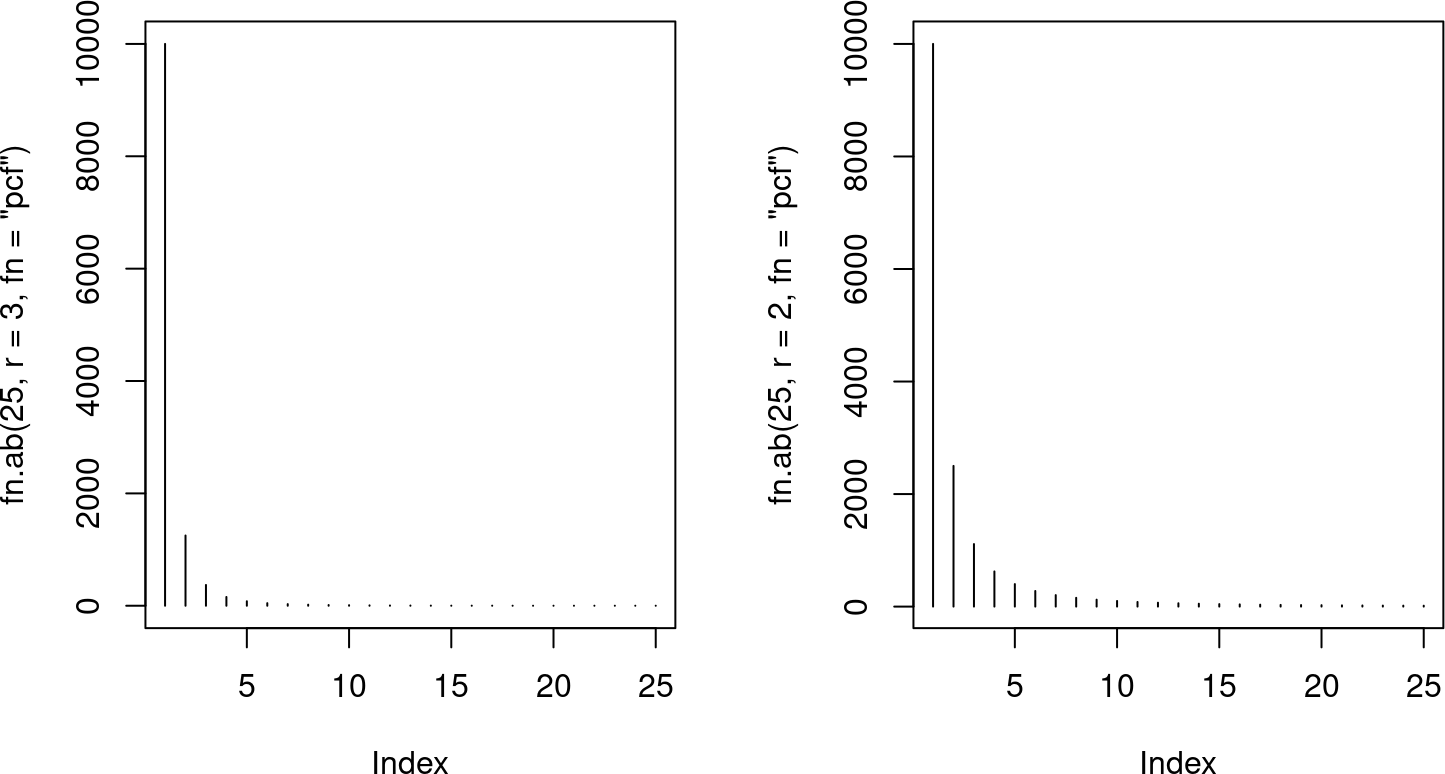Figure 2: Abundances simulated with fn.ab with fn=pcf

## 3.3 Decreasing fractional powers

$$fn.ab$$ with $$fn="dfp"$$ computes decreasing roots of the maximum frequency, $$h$$, according to:

$h^{1/i}, ~~ i=1..n$

table(fn.ab(25,fn="dfp"))
##
##     1     2     3     4     6    10    21   100 10000
##    12     5     2     1     1     1     1     1     1
par(mfrow=c(1,2))
plot(fn.ab(25,fn="dfp"),type="h")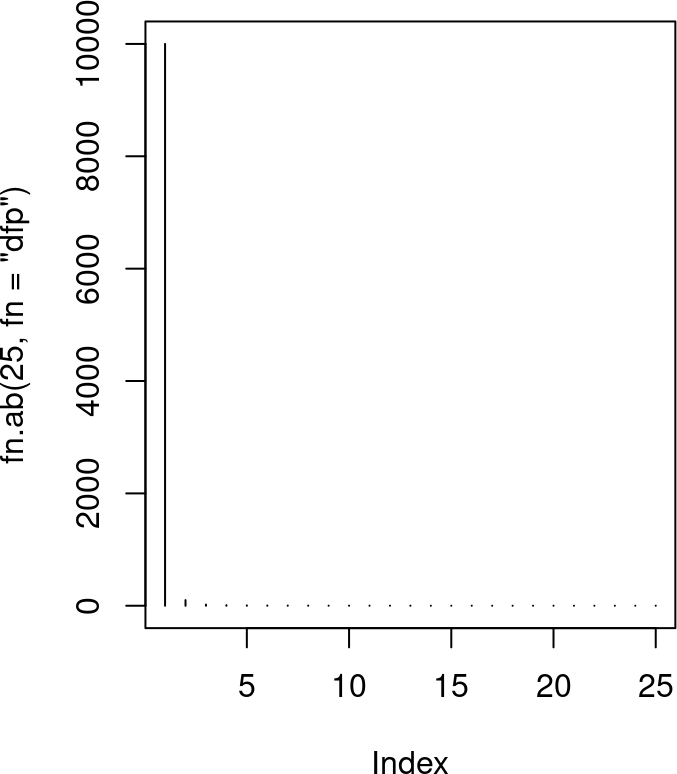Figure 3: Abundances simulated with fn.ab with fn=dpf

The figure and the previous table both show that this function is the one that generates the greatest distance between the dominant haplotype and the others.

To compare the profiles of the three functions, this figure plots the outputs of the functions with default parameters.

{rplot-fn3.2,fig.cap="Comparison of the data simulation functions"} par(mfrow=c(1,3)) plot(fn.ab(25,fn="pf"),type="h",main="fn.ab - pf") plot(fn.ab(25,r=3,fn="pcf"),type="h",main="fn.ab - pcf") plot(fn.ab(25,fn="dfp"),type="h",main="fn.ab - dpf")

A linear combination of results of the three functions provides greater flexibility.

ab <- 0.25*fn.ab(25,fn="pf")+0.75*fn.ab(25,r=3,fn="pcf")
table(ab)
## ab
##      1   1.75    2.5    3.5   4.75    7.5  12.25   19.5  33.75  60.75  112.5
##      8      3      1      1      1      1      1      1      1      1      1
## 216.25  429.5  902.5 2187.5  10000
##      1      1      1      1      1
plot(ab,type="h",main="Linear combination of results")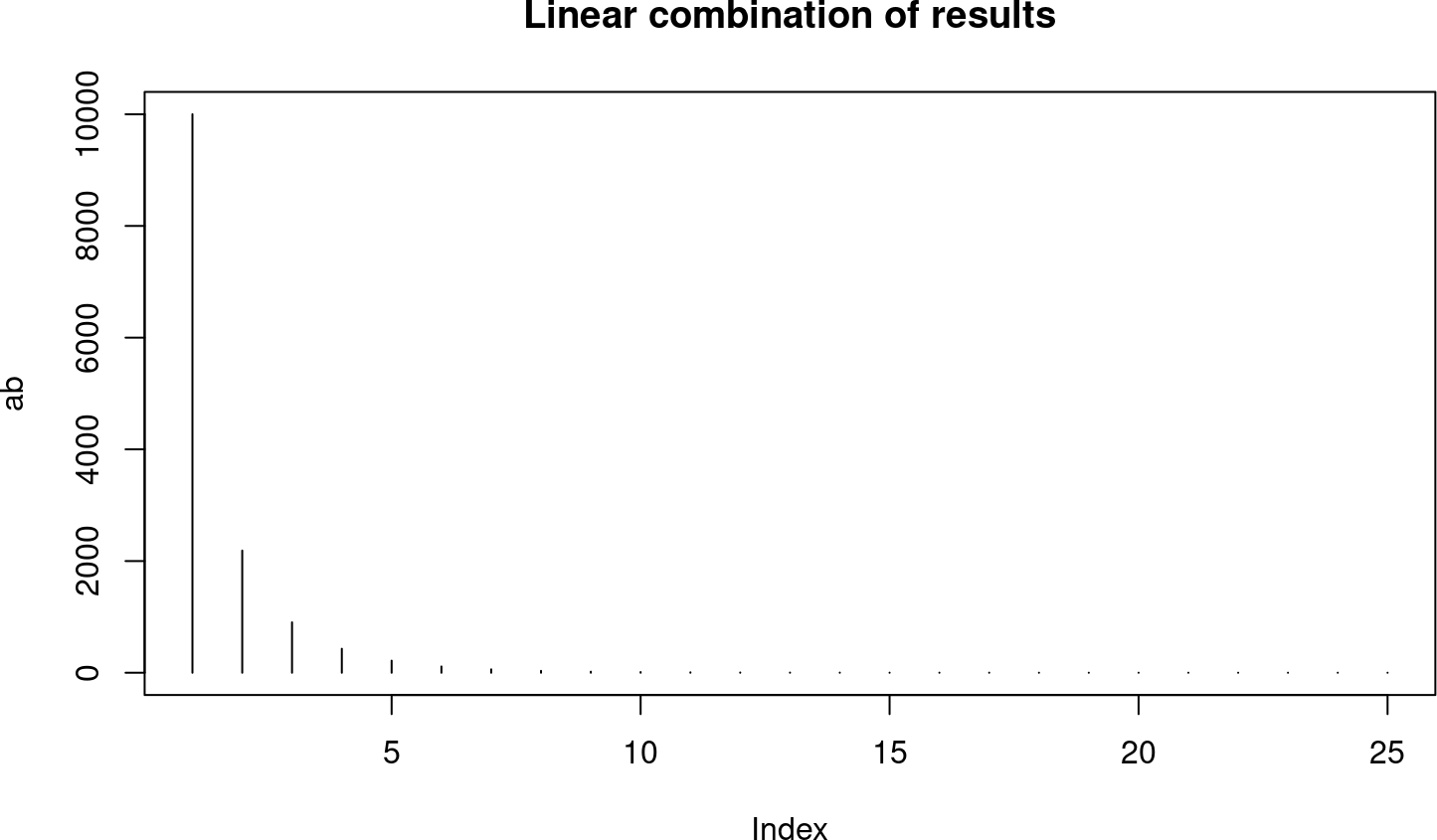Figure 4: Abundances simulated with a linear combination of the functions

ab <- 0.7*fn.ab(25,fn="pf")+0.3*fn.ab(25,fn="dfp")
table(ab)
## ab
##      1      2    3.4    6.9   13.9   27.9   55.5  110.1  219.6  439.3    878
##     12      1      1      1      1      1      1      1      1      1      1
## 1756.3   3530  10000
##      1      1      1
plot(ab,type="h",main="Linear combination of results")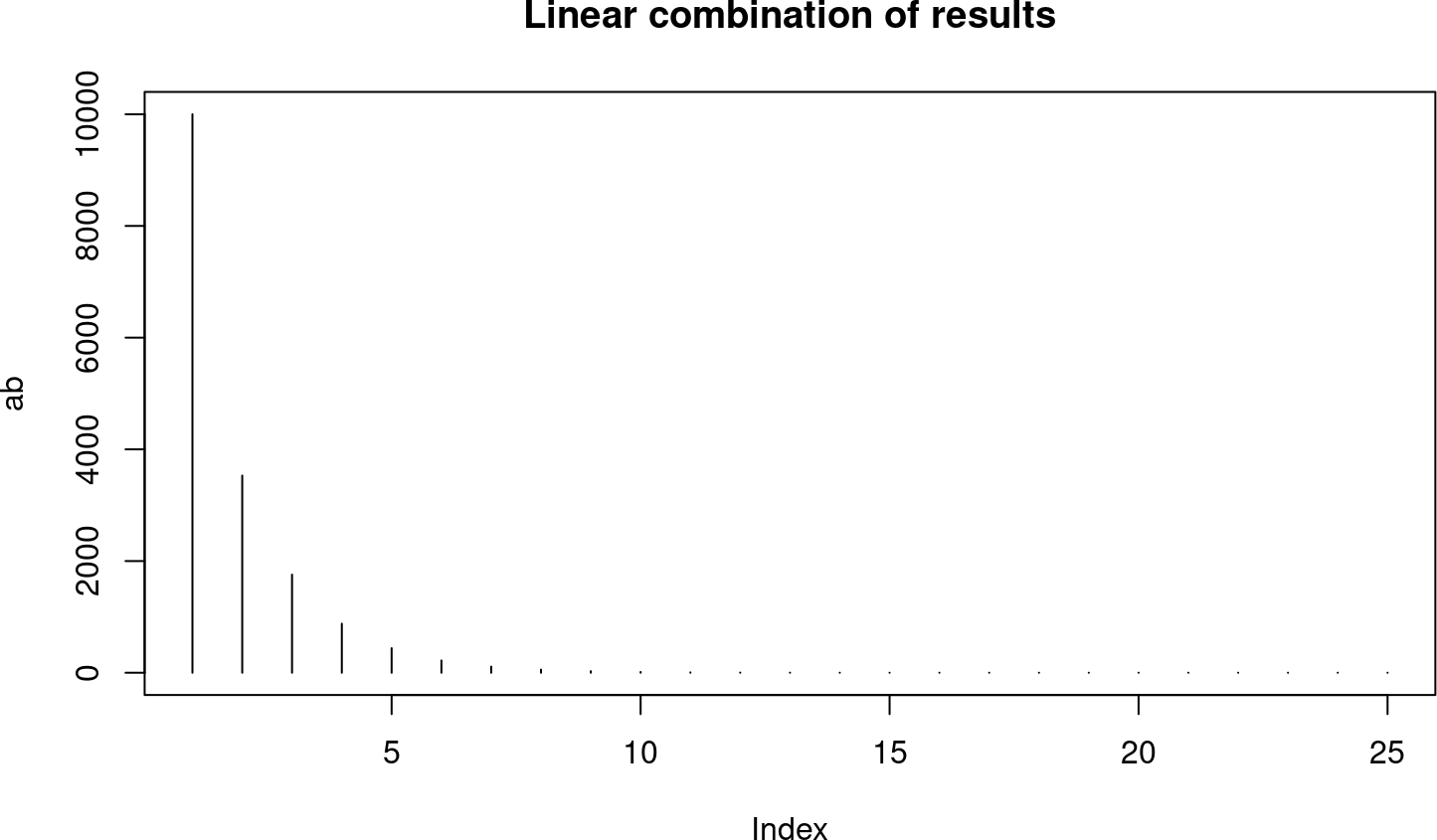Figure 5: Abundances simulated with a linear combination of the functions

## 3.4 Geometric sequence

Appropriate for the typical load of rare haplotypes observed in our experiments with the HCV quasispecies before the abundance filter. That is, the large number of very low fitness or defective haplotypes are best simulated by a geometric sequence with low values for the parameter. The geometric sequence is expressed as:

$p ~(1-p)^{k-1}, ~~ k=1..n, ~~ 0 < p < 1$

and is implemented in the function $$geom.series$$, taking two arguments: $$n$$, the number of frequencies to compute and $$p$$, the parameter of the geometric function.

This function is useful to simulate a broad spectrum of frequency profiles, from quasispecies with very prevalent haplotypes to the above-mentioned long queues of very low abundances, as illustrated in the next figure.

par(mfrow=c(1,2))
ab1 <- 1e5 * geom.series(100,0.8)
plot(ab1,type="h",main="Geometric series with p=0.8",cex.main=1)
ab2 <- 1e5 * geom.series(100,0.001)
plot(ab2,type="h",main="Geometric series with p=0.001",ylim=c(0,max(ab2)),
cex.main=1)